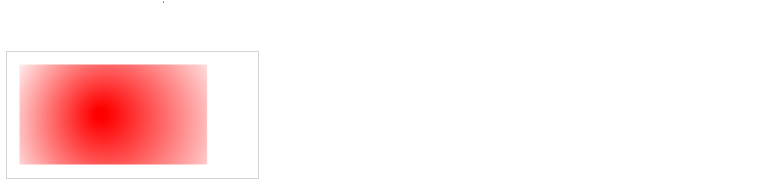# HTML Canvas Graphics

The HTML `<canvas>` element is used to draw graphics on a web page.

## What is HTML Canvas?​

The HTML `<canvas>` element is used to draw graphics, on the fly, via JavaScript.

The `<canvas>` element is only a container for graphics. You must use JavaScript to actually draw the graphics.

Canvas has several methods for drawing paths, boxes, circles, text, and adding images.

## Canvas Examples​

A canvas is a rectangular area on an HTML page. By default, a canvas has no border and no content.

The markup looks like this:

``<canvas id="myCanvas" width="300" height="100"></canvas>``
note

Always specify an `id` attribute (to be referred to in a script), and a `width` and `height` attribute to define the size of the canvas. To add a border, use the `style` attribute.

Example of an empty canvas:

``<canvas id="myCanvas" width="200" height="100" style="border:1px solid #000000;">  </canvas>``
note

Coordinates (0,0) defines the upper left corner of the canvas.

Output: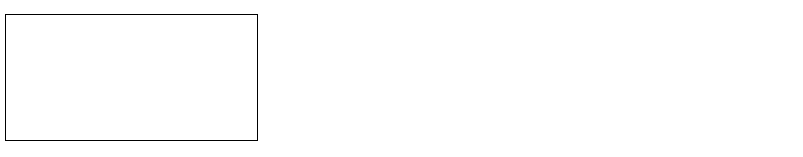After creating the rectangular canvas area, you must add JavaScript code to do the drawing.

Here are some examples.

### Draw a Line​

If you want to draw a straight line on the canvas, you can use the following two methods:

• moveTo(x,y): It is used to define the starting point of the line.

• lineTo(x,y): It is used to define the ending point of the line.

If you draw a line which starting point is (0,0) and the end point is (200,100), use the stroke method to draw the line.

``<script>      var c = document.getElementById("myCanvas");      var cctx = c.getContext("2d");      ctx.moveTo(0,0);      ctx.lineTo(200,100);      ctx.stroke();  </script>  ``

Output: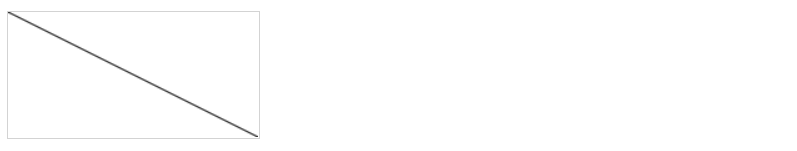### Draw a Circle​

If you want to draw a circle on the canvas, you can use the arc() method:

``arc(x, y, r, start, stop)``

To sketch circle on HTML canvas, use one of the ink() methods, like stroke() or fill().

``<script>      var c = document.getElementById("myCanvas");      var cctx = c.getContext("2d");      ctx.beginPath();      ctx.arc(95,50,40,0,2*Math.PI);      ctx.stroke();  </script>  ``

Output: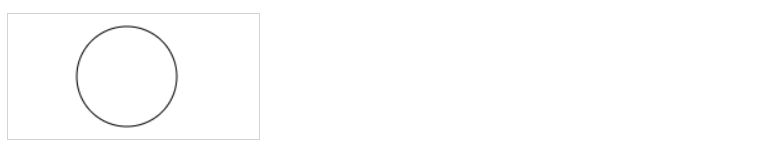### Draw a Text​

There are property and methods used for drawing text on the canvas.

• font property: It is used to define the font property for the text.
• fillText(text,x,y) method: It is used to draw filled text on the canvas. It looks like bold font.
• strokeText(text,x,y) method: It is also used to draw text on the canvas, but the text is unfilled.

Let's see fillText() method example.

``<script>      var c = document.getElementById("myCanvas");      var cctx = c.getContext("2d");      ctx.font = "30px Arial";      ctx.fillText("Hello World",10,50);  </script>  ``

Output: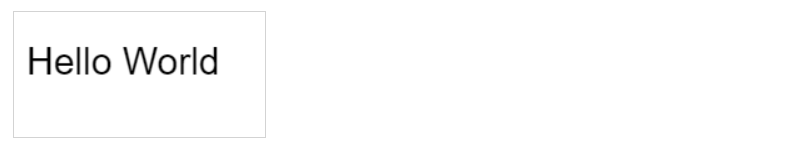Let's see strokeText() method example.

``<script>      var c = document.getElementById("myCanvas");      var cctx = c.getContext("2d");      ctx.font = "30px Arial";      ctx.strokeText("Hello World",10,50);  </script>  ``

Output: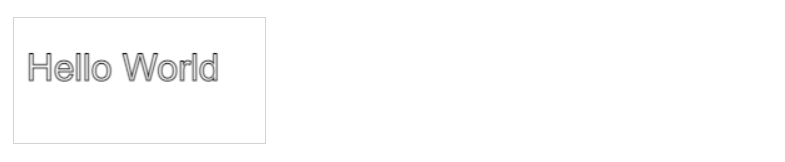``<script>      var c = document.getElementById("myCanvas");      var ctx = c.getContext("2d");            // Create gradient      var grd = ctx.createLinearGradient(0, 0, 200, 0);      grd.addColorStop(0, "red");      grd.addColorStop(1, "white");            // Fill with gradient      ctx.fillStyle = grd;      ctx.fillRect(10, 10, 150, 80);  </script>``

Output: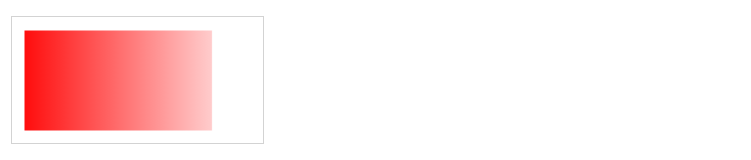``<script>      var c = document.getElementById("myCanvas");      var ctx = c.getContext("2d");            // Create gradient      var grd = ctx.createRadialGradient(75, 50, 5, 90, 60, 100);      grd.addColorStop(0, "red");      grd.addColorStop(1, "white");            // Fill with gradient      ctx.fillStyle = grd;      ctx.fillRect(10, 10, 150, 80);  </script>``